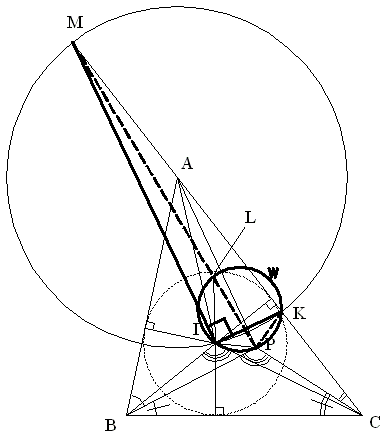## Problem 1 from the IMO 2006

Here is Problem 1 from the IMO 2006:

Let ABC be a triangle with incenter I. A point P in the interior of the triangle satisfies ∠PBA + ∠PCA = ∠PBC + ∠PCB. Show that AP ≥ AI, and that equality holds if and only if P = I.

The solution below is by Vo Duc DienChase the angles:

∠BIC = 180 - ½ (∠ABC + ∠ACB).

∠BPC = 180 - (∠PBC + ∠PCB).

The problem gives us

 ∠PBA + ∠PCA = ∠PBC + ∠PCB = ½ (∠ABC + ∠ACB).

Therefore, ∠BPC = ∠BIC.

Draw a circle with center at A that passes through point I and intersects AC at K.

 (1) ∠MPK = 360° - ∠BPC - ∠MPB - ∠KPC

But

 ∠BPC = ∠BIC, ∠MPB = ∠MIB - ∠IMP - ∠IBP, ∠KPC = ∠KIC + ∠IKP + ∠ICP, ∠IBP = ½ ∠ABC - ∠PBC, ∠ICP = ∠PCB - ½ ∠ACB.

So that (1) becomes

 ∠MPK = 360° - ∠BIC - ∠MIB + ∠IMP + ∠IBP - ∠KIC - ∠IKP - ∠ICP = 360° - ∠BIC - ∠MIB + ∠IMP + ½∠ABC - ∠PBC - ∠KIC - ∠IKP + ½∠ACB - ∠PCB.

And since ½(∠ABC + ∠ACB ) = ∠PBC + ∠PCB,

 ∠MPK = (360° - ∠BIC - ∠MIB - ∠KIC) + ∠IMP - ∠IKP ∠MIK = 360° - ∠BIC - ∠MIB - ∠KIC = 90° ∠MPK = 90° + ∠IMP - ∠IKP = 90° + ∠IMP - ∠ILP

Further, ∠ILP > ∠IMP implies ∠IMP - ∠ILP < 0, or

∠MPK < 90°.

Therefore, point P is outside the circle with center A implying AP > AI.

(Note: a shorter solution is available elsewhere.)